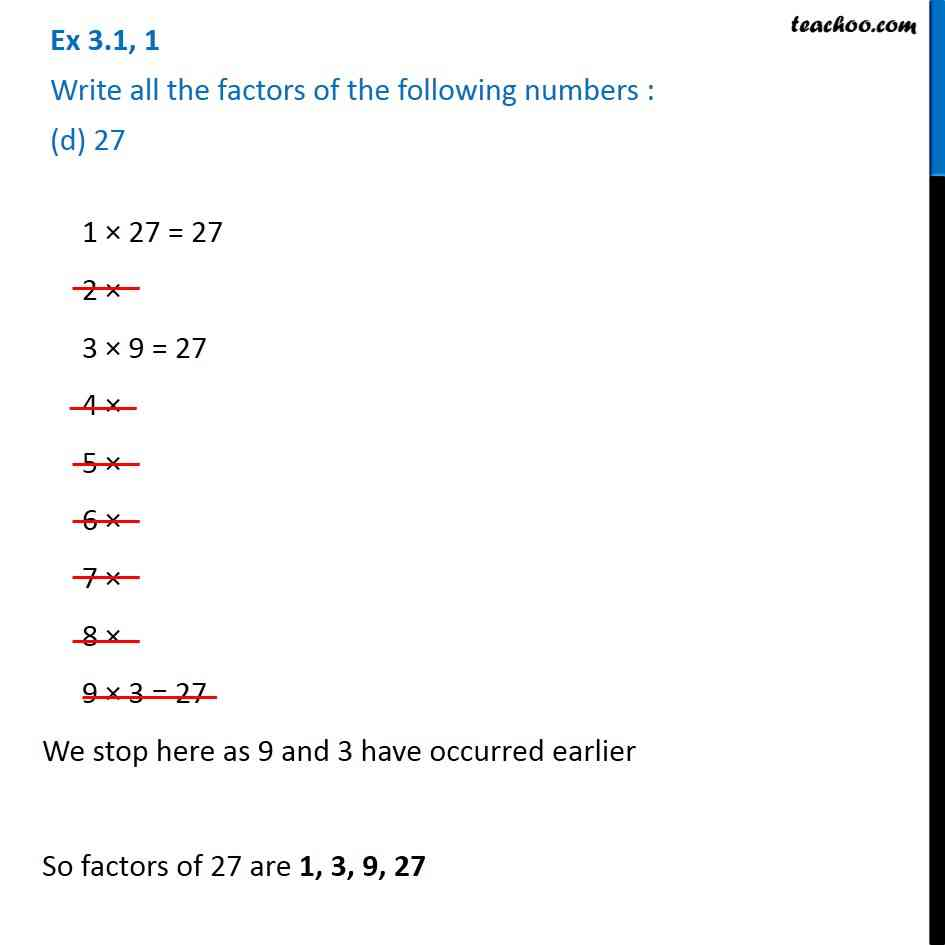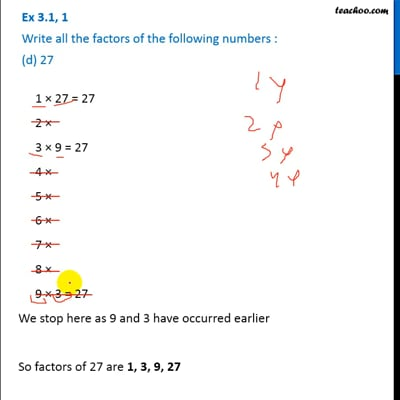Ex 3.1

Chapter 3 Class 6 Playing with Numbers
Serial order wiseThis video is only available for Teachoo black users

Introducing your new favourite teacher - Teachoo Black, at only ₹83 per month

### Transcript

Ex 3.1, 1 Write all the factors of the following numbers : (d) 27 1 × 27 = 27 2 × 3 × 9 = 27 4 × 5 × 6 × 7 × 8 × 9 × 3 = 27 We stop here as 9 and 3 have occurred earlier So factors of 27 are 1, 3, 9, 27 1 × 27 = 27 2 × 3 × 9 = 27 4 × 5 × 6 × 7 × 8 × 9 × 3 = 27 We stop here as 9 and 3 have occurred earlier So factors of 27 are 1, 3, 9, 27